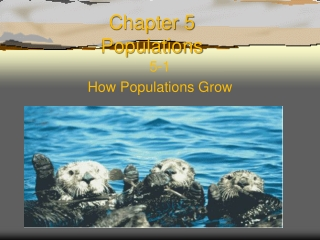Download PresentationChapter 5 Populations

# Chapter 5 Populations - PowerPoint PPT PresentationDownload Presentation## Chapter 5 Populations

- - - - - - - - - - - - - - - - - - - - - - - - - - - E N D - - - - - - - - - - - - - - - - - - - - - - - - - - -
##### Presentation Transcript

1. Chapter 5Populations 5-1 How Populations Grow

2. KWL • What are three things you want to know about population growth? • What do you know about population growth?

3. How Populations Grow • Characteristics of Populations • Three important characteristics of a population • geographic distribution • Density • growth rate

4. Geographic Distribution Geographic Distribution • Geographic distribution, or range, is a term that describes the area inhabited by a population.

5. Population Density • Population density is the number of individuals per unit area. • The population of saguaro cactus in the desert plant community has a low density, whereas other plants in that community have a relatively high density.

6. Populations Growth • Population Growth • Three factors can affect population size: • number of births • the number of deaths • the number of individuals that enter or leave the population. * Simply put, a population will increase or decrease in size depending on how many individuals are added to it or removed from it

7. Immigration & Emmigration • Immigration • the movement of individuals into an area, is another factor that can cause a population to grow. • Emigration • the movement of individuals out of an area, can cause a population to decrease in size.

8. Word Origin • Immigration is formed from the Latin prefix in-, meaning “in,” and migrare, meaning “to move from one place to another. • If the Latin prefix e- means “out,” then which of the following means “migration out”? • A.) emigration • B.) migration • C.) Immigration

9. Exponetial Growth • Exponential Growth • If a population has abundant space and food, and is protected from predators and disease, then organisms in that population will multiply and the population size will increase.

10. Exponential Growth Exponential growth occurs when the individuals in a population reproduce at a constant rate. Under ideal conditions with unlimited resources, a population will grow exponentially.

11. Checkpoint Which of the following is NOT a condition for a population to reach exponential growth? A.) presence of unlimited resources B.) Absence of predation and disease C.) movement of individuals out of the population

12. Logistic Growth • Logistic growth occurs when a population's growth slows or stops following a period of exponential growth. • As resources become less available, the growth of a population slows or stops. The general, S-shaped curve of this growth pattern, called logistic growth

13. Carrying Capacity • Carrying Capacity  The number or the largest number of individuals that a given environment can support.

14. List three characteristics that are used to describe a population. What factors can change a population's size? What is the difference between exponential growth and logistic growth?

15. Using Graphic Organizers • Draw a concept map that shows how populations grow. Include the following terms: exponential growth, logistic growth, birthrate, death rate, immigration, emigration. Add any other terms that you think are useful to complete the map